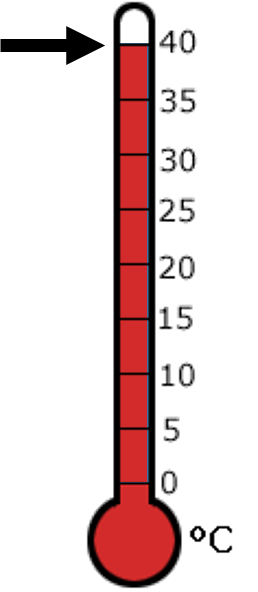1
visibility

What is the temperature shown on the given thermometer?• \$latex 50^\circ C\$

• \$latex 40^\circ C\$

• \$latex 20^\circ C\$

• \$latex 30^\circ C\$

The correct answer is  40°C.

Solution:

First, we find the point where the thermometer changes from red to white.Then, we look at the number to the right.

The temperature is  40°C  as desired.